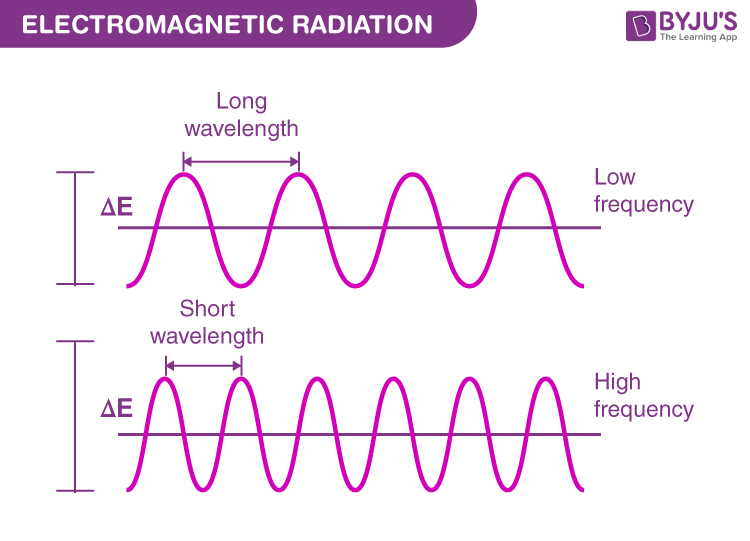# Relation between Frequency and Wavelength

## What is Frequency?

Frequency is defined as the number of oscillations of a wave per unit time being, measured in hertz(Hz). The frequency is directly proportional to the pitch. Humans can hear sounds with frequencies ranging between 20 – 20000 Hz. Sounds with frequencies over the human ears range are known as ultrasound, and sounds with frequencies less than the audible range are known as infrasound.You need to know what Wavelength and frequency mean to understand their relationship.

## What is Wavelength?

All points on a wave oscillate, i.e., all points on a wave exhibit a type of regular change in a particular value. For instance, when you make a wave by wiggling up and down, the rope molecules repeatedly move up and down. When you consider an electromagnetic wave, the value of magnetic and electric fields keeps changing because of the wave at a point. If the wave is not a short pulse, the value of electric and magnetic fields keep changing at any point. For example, two points on a wave that attain their maximum value oscillate simultaneously.

Wavelength is defined as the distance between two most near points in phase with each other. Hence, two adjacent peaks or troughs on a wave are separated by a distance of a single complete wavelength. Mostly, we use the letter lambda (λ) to describe the wavelength of a wave.

## Relation between Frequency and Wavelength

You can create a wave having some frequency on a rope and then gradually make it faster. During this, you will notice that as the wavelength becomes shorter. Therefore, the relation between frequency and wavelength does exist, so let’s find out what exactly this relationship is.

Another quantity is Period (T) which can be used to describe a wave. It is defined as the time taken to complete one oscillation. Because frequency determines the number of times a wave oscillates and it can be expressed as,
f = 1 / t

Every point on the wave returns to the same value after one period since a wave undergoes one oscillation during one period. This occurs because every oscillation session travels a wavelength distance from one period to finish.

The wave speed (v) is defined as the distance travelled by a wave per unit of time. If considering that the wave travels a distance of one wavelength in one period,

ν=λ/T

As we know that T = 1/f, hence we can express the above equation as,

V = f λ

The wave speed is equal to the product of its frequency and wavelength, and this implies the relationship between frequency and wavelength.

Learn the relationship between velocity and wavelength and the relationship between amplitude and frequency by clicking on the links below:

## Frequently Asked Questions – FAQs

Q1

### What is the frequency?

Frequency is defined as the number of oscillations of a wave per unit of time and is measured in hertz(Hz).
Q2

### What is the connection between frequency and pitch?

The frequency is directly proportional to the pitch.
Q3

### What is the audible frequency range of humans?

Humans can hear sounds with frequencies ranging between 20 – 20000 Hz.
Q4

### What is a wavelength?

Wavelength is defined as the distance between two nearest points in phase with each other.
Q5

### What is the relationship between frequency and wavelength?

The wave speed is equal to the product of its frequency and wavelength.
V = f λ

Stay tuned with BYJU’S to learn more about waves, ultrasound waves, nature, and properties with the interactive video lessons.

Test Your Knowledge On Frequency And Wavelength!